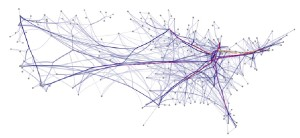# What Math Skills do I need for a Game Design Degree?

Game design is one of the most popular subjects in computer science, and while it requires most of the same math concepts as other areas of programming, there are a few concepts related to graphics and problem solving that game developers need more than others. By far, the most important math skills needed for game design are related to 3-D graphics and animation, and these skills are based on matrix math and linear algebra, as well as logic and discrete math. Because time and motion are involved in a game loop, programmers also use continuous math concepts from calculus and trigonometry. These continuous math skills also apply to the physics needed to make animations look realistic.

### Most Important Math Concepts for Game ProgrammersAll areas of math are useful to game programmers, but one skill that is particularly useful deals with graph algorithms. A graph is a set of objects, called nodes, that are related to one another by the edges that form paths between them. For example, a graph can be used to model a street grid. In this case, the nodes represent locations on the map, and the edges represent the streets connecting the locations. The most important graph algorithms for game designers are the search functions that find the shortest path between two nodes. These functions are used to find paths for game characters on a tile-based map when there are moving objects blocking some paths. They’re also used to pre-process a map and store the shortest paths in memory so that retrieving them doesn’t require a looping algorithm and can be performed in what’s called constant time.

In game design college, students learn about the same data structures and algorithms as computer science students, but they focus more on graphics programming and game production. The math skills needed for game design aren’t necessarily difficult, but it’s up to the student to become proficient in these areas. Linear algebra is one of the easier college math subjects, but students who are really interested in using the concepts taught in linear algebra will be more imaginative when applying them to games. The basic way linear algebra is used in 3-D programming is by rotating, scaling and moving objects in a 3-D world or performing these transformations on the camera itself. It’s also used to transform the 3-D world to the flat coordinate system of the computer screen. A matrix is sort of like a plane or a cage that remaps all the points of an object according to how it’s bent or shaped.

### Advanced Math for Game Design

Some of the more difficult concepts are related to discrete math and algorithm design. It’s not necessary to have a deep, profound understanding of algorithm design unless you want to be a researcher, but being able to analyze the time complexity of a function is a fundamental skill. Discrete and continuous math concepts are very useful in this area, especially the math related to summations, recursion and integral calculus, which allows you to approximate the sum of a series.

With mobile and Web-based games becoming more and more popular, there is a growing demand for game designers with solid skills. If you have an interest in designing the physics and graphics for video games, keep learning about the math skills needed for game design.